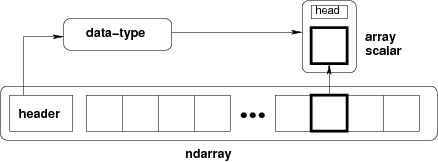# NumPy - Ndarray Object

The most important object defined in NumPy is an N-dimensional array type called ndarray. It describes the collection of items of the same type. Items in the collection can be accessed using a zero-based index.

Every item in an ndarray takes the same size of block in the memory. Each element in ndarray is an object of data-type object (called dtype).

Any item extracted from ndarray object (by slicing) is represented by a Python object of one of array scalar types. The following diagram shows a relationship between ndarray, data type object (dtype) and array scalar type −An instance of ndarray class can be constructed by different array creation routines described later in the tutorial. The basic ndarray is created using an array function in NumPy as follows −

```numpy.array
```

It creates an ndarray from any object exposing array interface, or from any method that returns an array.

```numpy.array(object, dtype = None, copy = True, order = None, subok = False, ndmin = 0)
```

The above constructor takes the following parameters −

Sr.No. Parameter & Description
1

object

Any object exposing the array interface method returns an array, or any (nested) sequence.

2

dtype

Desired data type of array, optional

3

copy

Optional. By default (true), the object is copied

4

order

C (row major) or F (column major) or A (any) (default)

5

subok

By default, returned array forced to be a base class array. If true, sub-classes passed through

6

ndmin

Specifies minimum dimensions of resultant array

Take a look at the following examples to understand better.

## Example 1

```import numpy as np
a = np.array([1,2,3])
print a
```

The output is as follows −

```[1, 2, 3]
```

## Example 2

```# more than one dimensions
import numpy as np
a = np.array([[1, 2], [3, 4]])
print a
```

The output is as follows −

```[[1, 2]
[3, 4]]
```

## Example 3

```# minimum dimensions
import numpy as np
a = np.array([1, 2, 3,4,5], ndmin = 2)
print a
```

The output is as follows −

```[[1, 2, 3, 4, 5]]
```

## Example 4

```# dtype parameter
import numpy as np
a = np.array([1, 2, 3], dtype = complex)
print a
```

The output is as follows −

```[ 1.+0.j,  2.+0.j,  3.+0.j]
```

The ndarray object consists of contiguous one-dimensional segment of computer memory, combined with an indexing scheme that maps each item to a location in the memory block. The memory block holds the elements in a row-major order (C style) or a column-major order (FORTRAN or MatLab style).